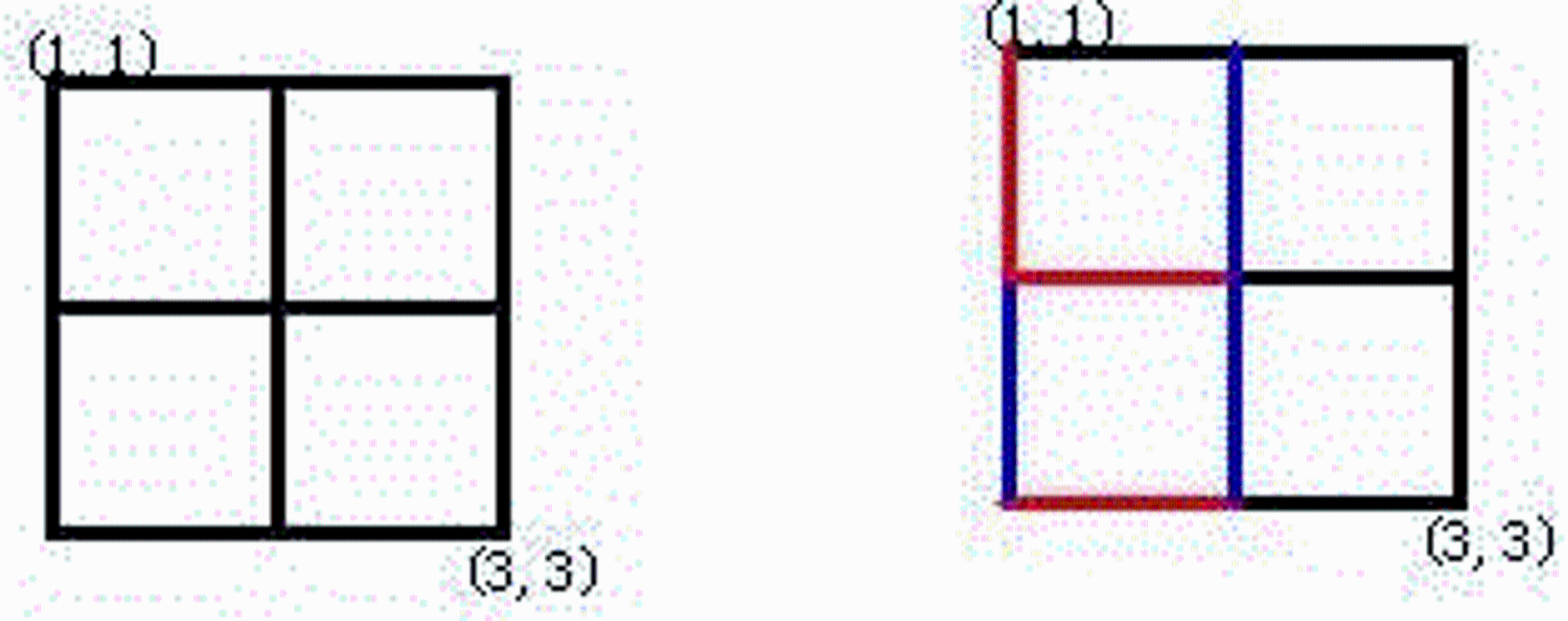V1

2022/10/22阅读：34主题：凝夜紫

# 前置知识

AcWing 1250. 格子游戏：二维坐标的并查集裸替，主要考察二维坐标转一维。

AcWing 1252. 搭配购买：使用并查集把相同集合的多个物品看做一个整体，方便使用01背包。

AcWing 237. 程序自动分析：带有离散化的并查集。

AcWing 238. 银河英雄传说：维护额外信息（距离和个数）的并查集。

AcWing 239. 奇偶游戏：综合应用。带有边权的并查集和带有扩展域的并查集，同时也应用了离散化。

# AcWing 1250. 格子游戏

Alice和Bob玩了一个古老的游戏：首先画一个n×n的点阵（下图n=3）。接着，他们两个轮流在相邻的点之间画上红边和蓝边：直到围成一个封闭的圈（面积不必为1）为止，“封圈”的那个人就是赢家。因为棋盘实在是太大了，他们的游戏实在是太长了！他们甚至在游戏中都不知道谁赢得了游戏。于是请你写一个程序，帮助他们计算他们是否结束了游戏？

1≤n≤200，
1≤m≤24000

``3 51 1 D1 1 R1 2 D2 1 R2 2 D``

``4``

## Code

``#include <iostream>#include <cstring>using namespace std;const int N = 40010, M = 24010;int n, m;int p[N], res;int find(int x) {    if (p[x] != x) p[x] = find(p[x]);    return p[x];}// 二维点坐标变成一维int get(int x, int y) {    return x * n + y;}int main() {    cin >> n >> m;    for (int i = 0; i <= n * n; i ++ ) p[i] = i;    for (int i = 1; i <= m; i ++ ) {        int x, y;        char t;        cin >> x >> y >> t;        // 二维转一维 坐标必须从0开始 不然不满足公式        x -- , y -- ;        int a = get(x, y);         int b;        if (t == 'D') b = get(x + 1, y);        else b = get(x, y + 1);        int pa = find(a), pb = find(b);        // 两个点在同一集合 则连边后形成封闭矩阵        if (pa == pb) {            res = i;            break;        }        p[pa] = pb; // 不在同一集合 合并两条边    }    if (!res) puts("draw");    else cout << res << endl;    return 0;}``

# AcWing 1252. 搭配购买

Joe觉得云朵很美，决定去山上的商店买一些云朵。商店里有n朵云，云朵被编号为1,2,…,n并且每朵云都有一个价值。但是商店老板跟他说，一些云朵要搭配来买才好，所以买一朵云则与这朵云有搭配的云都要买。但是Joe的钱有限，所以他希望买的价值越多越好。

1≤n≤10000,
0≤m≤5000,
1≤w≤10000,
1≤ci≤5000,
1≤di≤100,
1≤ui,vi≤n

``5 3 103 103 103 105 10010 11 33 24 2``

``1``

（买云朵的故事真是太浪漫啦~~）

## Code

``#include <iostream>#include <cstring>using namespace std;const int N = 10010;int p[N], w[N], v[N], f[N];int n, m, money;int find(int x) {    if (p[x] != x) p[x] = find(p[x]);    return p[x];}int main() {    cin >> n >> m >> money;    for (int i = 1; i <= n; i ++ ) p[i] = i;    for (int i = 1; i <= n; i ++ ) cin >> v[i] >> w[i];        while (m -- ) {        int a, b;        cin >> a >> b;        int pa = find(a); int pb = find(b);        // 合并一个集合里的云朵 价格和价值 别忘记合并        if (pa != pb) {            w[pa] += w[pb];            v[pa] += v[pb];            p[pb] = pa;        }    }    // 01背包模板    for (int i = 1; i <= n; i ++ ) {        if (p[i] == i)            for (int j = money; j >= v[i]; j -- )                f[j] = max(f[j], f[j - v[i]] + w[i]);    }    cout << f[money] << endl;    return 0;}``

# AcWing 237. 程序自动分析

1≤n≤10^5
1≤i,j≤10^9

``````2
1 2 1
1 2 0
2
1 2 1
2 1 1
``````

``NOYES``

## Code

（离散化不是本文重点，因此不做过多讲述，代码中有注释。）

``#include <iostream>#include <cstring>#include <unordered_map>using namespace std;const int N = 2000010;int n, m;int p[N];unordered_map<int, int> S; // 离散化hash表struct Query {    int x, y, e;}query[N];// 超级简单的不保序离散化// 将单个数据范围在1~10^9 压缩到 单个数据范围在1~10^6int get(int x) {    // 如果x不在哈希表中 就给它一个映射值。    if (S.count(x) == 0) S[x] = ++ n;    return S[x]; // 返回x在哈希表的位置}int find(int x) {    if (p[x] != x) p[x] = find(p[x]);    return p[x];}int main() {    int T;    cin >> T;    while (T -- ) {        cin >> m;        n = 0;        S.clear();        for (int i = 0; i < m; i ++ ) {            int a, b, c;            scanf("%d%d%d", &a, &b, &c);            query[i] = {get(a), get(b), c};        }        for (int i = 1; i <= n; i ++ ) p[i] = i;        // 合并 所有的 相等约束条件        for (int i = 0; i < m; i ++ ) {            if (query[i].e == 1) {                int pa = find(query[i].x);                int pb = find(query[i].y);                p[pa] = pb;            }        }        // 检查不等条件        bool flag = false;        for (int i = 0; i < m; i ++ ) {            if (query[i].e == 0 ) {                int pa = find(query[i].x);                int pb = find(query[i].y);                // 在同一个集合说明相等 与 不等条件冲突                if (pa == pb) {                     flag = true;                    break;                }            }        }        if (flag) puts("NO");        else puts("YES");    }    return 0;}``

# AcWing 238. 银河英雄传说

1. `M i j`，表示让第i号战舰所在列的全部战舰保持原有顺序，接在第j号战舰所在列的尾部。
2. `C i j`，表示询问第i号战舰与第j号战舰当前是否处于同一列中，如果在同一列中，它们之间间隔了多少艘战舰。

N≤30000,T≤500000

``4M 2 3C 1 2M 2 4C 4 2``

``-11``

## 题目分析

d[x]：表示集合中的元素x到它的根节点p[x]的距离。

## Code

``#include <iostream>#include <cstring>using namespace std;const int N = 300010;int n, m, p[N], sz[N], d[N];// 维护距离的并查集模板int find(int x) {    if (p[x] != x) {        int root = find(p[x]); // 先递归到根节点        d[x] += d[p[x]];  // 根节点开始累加        p[x] = root;    }    return p[x];}int main() {    cin >> m;    // 初始化 每个节点到自身的距离为0     // 初始化 每个集合中的数据个数为1 也就是根节点自己    for (int i = 1; i <= N; i ++ )        p[i] = i, d[i] = 0, sz[i] = 1;    while (m -- ) {        int a, b;        char t;        cin >> t >> a >> b;        int pa = find(a), pb = find(b);        if (t == 'M') {            // 当两个数据不在一个集合 则合并            if (pa != pb) {                // 合并 且维护信息                p[pa] = pb;                d[pa] = sz[pb];                sz[pb] += sz[pa];            }        } else {            if (pa != pb) cout << -1 << endl;            else {                // 注意输出的时候 因为减一的关系 可能会负                // 但是有解情况 最小是0 也就是自己到自己                cout << max(abs(d[a] - d[b]) - 1, 0) << endl;            }        }    }    return 0;}``

# AcWing 239. 奇偶游戏

N≤109,M≤5000

``1051 2 even3 4 odd5 6 even1 6 even7 10 odd``

``3``

## 题目分析

1. S[L,R]有偶数个1，等价于sum[L-1]与sum[R]的奇偶性相同。例如，示例中有S[1,3]有奇数个1，S[4,6]有偶数个1。而区间S[4,6]又可以拆分成sum和sum，并且他们两个奇偶性应该相同，而sum就是区间S[1,3]是奇数，所以sum就是区间S[1,6]也应该是奇数。

2. S[L,R]有奇数个1，等价于sum[L-1]与sum[R]的奇偶性不同。与上面同理。

1. 如果x1和x2的奇偶性相同，x2和x3奇偶性也相同，那么x1和x3奇偶性相同；

2. 如果x1和x3的奇偶性相同，x2和x3奇偶性不同，那么x1和x3奇偶性不同；

3. 如果x1和x2的奇偶性不同，x2和x3奇偶性也不同，那么x1和x3的奇偶性相同；

### 带边权的并查集

1. 对于给定的每个区间，我们判断sum[L]和sum[R]是否属于一个集合（奇偶性关系已知）。

2. 属于一个集合的话，判断他们在集合内的奇偶关系与给出的奇偶关系是否相同，如果不相同，说明出现矛盾，小A说谎了。

3. 如果不属于一个集合，则将两个数据合并。

## Code

### 带边权的并查集

1. x到x的原根节点p[x]，这部分就是d[x]；

2. x的原根节点p[x]到y，也就是`d[p[x]]`我们是不知道的，但是我们知道合并后的结果，假设合并后的结果是t，那么就有`t=d[x]^d[y]^d[p[x]]`，如此，我们就推出了`d[p[x]]=d[x]^d[y]^t`

3. y到根节点p[y]，这部分就是d[y]；

``#include <iostream>#include <cstring>#include <unordered_map>using namespace std;const int N = 20010;int n, m;int p[N], d[N];unordered_map<int, int> S;int find(int x) {    // 带权并查集的路径压缩 必须注意细节    // 一定 先计算边权 再压缩    if (p[x] != x) {        int root = find(p[x]);        d[x] ^= d[p[x]];        p[x] = root;    }    return p[x];}int get(int x) {    if (S.count(x) == 0) S[x] = ++ n;    return S[x];}int main() {    cin >> n >> m;    n = 0;    for (int i = 0; i < N; i ++ ) p[i] = i;    int res = m; // 没有矛盾输出问题的数量    for (int i = 0; i < m; i ++ ) {        int a, b;        string c;        cin >> a >> b >> c;        // 获取离散化后的sum[L-1]和sum[R]        a = get(a - 1), b = get(b);        int t = 0; // 0表示偶数个1        if (c == "odd") t = 1; // 1表示有奇数个1        int pa = find(a), pb = find(b);        // 如果两个数据的奇偶关系已知        if (pa == pb) {            // 判断他们的奇偶关系 是否和本次数据相同            if ((d[a] ^ d[b]) != t) {                res = i;                break;            }        } else {            p[pa] = pb;            // 并查集到根            d[pa] = d[a] ^ d[b] ^ t;        }    }    cout << res << endl;    return 0;}``

### 带扩展域的并查集

``#include <iostream>#include <cstring>#include <unordered_map>using namespace std;const int N = 20010, Base = N / 2;int n, m;int p[N], d[N];unordered_map<int, int> S;int find(int x) {    if (p[x] != x) p[x] = find(p[x]);    return p[x];}int get(int x) {    if (S.count(x) == 0) S[x] = ++ n;    return S[x];}int main() {    cin >> n >> m;    n = 0;    for (int i = 0; i < N; i ++ ) p[i] = i;    int res = m; // 没有矛盾输出问题的数量    for (int i = 0; i < m; i ++ ) {        int a, b;        string c;        cin >> a >> b >> c;        // 获取离散化后的sum[L-1]和sum[R]        a = get(a - 1), b = get(b);        int t = 0; // 0表示偶数个1        // 假如给出数据是X和Y是偶数关系        // 则有Xodd和Yodd 在一个集合 Xeven和Yeven在一个集合        if (c == "even") {            // 如果Xodd和Yeven在一个集合 一定矛盾            if (find(a + Base) == find(b)) {                res = i;                break;            }            p[find(a)] = p[find(b)]; // Xodd和Yodd合并到一个集合            p[find(a + Base)] = p[find(b + Base)]; // Xeven和Yeven合并到一个集合        } else {            // 反之，则相反            if (find(a) == find(b)) {                res = i;                break;            }            p[find(a)] = p[find(b + Base)]; // Xeven和Yodd在一个集合            p[find(a + Base)] = p[find(b)]; // Xodd 和 Yeven在一个集合        }    }    cout << res << endl;    return 0;}``

ENDV1